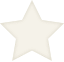# 不走运子弟纪事河上第十三家 著

𘢁𘢅𘢈𘢃|𘢋𘢋𘢅阅文总点击|𘢌会员周点击|𘢁总推荐|𘢌周推荐

##### 我要评价本作乃是一个短篇故事合集。虽然也有一些贯穿所有短篇的主人公，但是每个短篇故事都是可以独立开来阅读的。
这些短篇故事的共同点就是都是在讽刺一些玄幻小说中的定律规则yy点。
<<<<<<<<<<<<<<<<<<<<<<<<<<<<<<<<<<<<<<<<<<<<<<<<<<<<<<<<<<
希望读者可以通过阅读这些短篇故事，保持愉快的心情，那就是在下的功德无量了。

0

0

100起点币=100点粉丝值

• 作品总数

1
• 累计字数

0.82万
• 创作天数

29